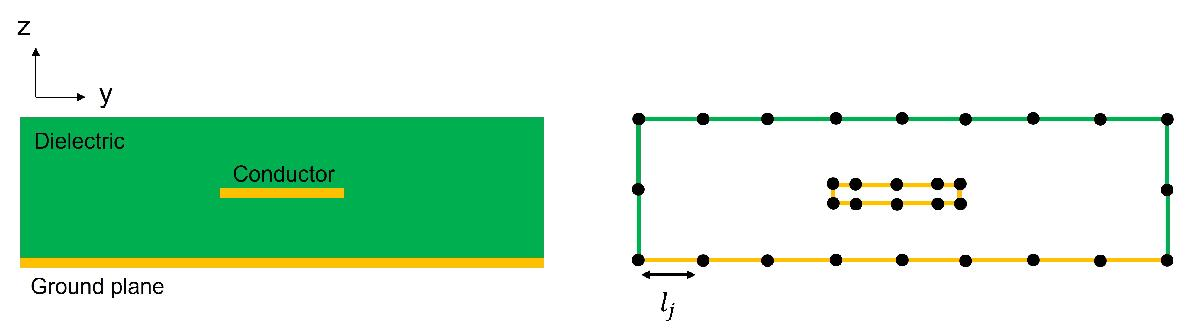## 2-D Field Solver

The 2-D field solver in RF PCB Toolbox™ allows you to model and analyze the cross-sections of multiconductor transmission lines in a multilayered dielectric above a ground plane like a microstrip line. For more detailed information, see 

The four primary transmission line parameters are the resistance R, the inductance L, the conductance G and the capacitance C. You can obtain these parameters by applying a total charge on the conductor-to-dielectric interfaces and a polarization charge on the dielectric-to-dielectric interfaces and solving the electrostatic field created. The 2D field solver uses pulse approximation for the total charge σT and discretizes the contours of the conductor-to-dielectric and dielectric-to-dielectric interfaces using straight line segments. The density of the segments are controlled by the distribution of nodes along these contours. The figure shows the discretization for a single conductor transmission line in a single layered dielectric above a ground plane.At any point p in the YZ plane and above the ground plane, the potential ϕ is due to the combination of σT

and the image of σT about the ground plane.

`$\varphi \left(p\right)=\frac{1}{2\pi {e}_{0}}\sum _{j=1}^{J}\int {\sigma }_{T}\left({p}^{\text{'}}\right)\left(\frac{|p-{\stackrel{^}{p}}^{\text{'}}|}{|p-{p}^{\text{'}}|}\right)dl\text{'}$`

where lj is the contour of jth interface in the YZ plane, dl' is the differential element of length at p' on lj and $\stackrel{^}{p}\text{'}$ is the image of p' about the ground plane.

The electric field is then given by φ

`$E\left(p\right)=\frac{1}{2\pi {e}_{0}}\sum _{j=1}^{J}\int {\sigma }_{T}\left({p}^{\text{'}}\right)\left(\frac{p-{p}^{\text{'}}}{{|p-{p}^{\text{'}}|}^{2}}-\frac{p-{\stackrel{^}{p}}^{\text{'}}}{{|p-{\stackrel{^}{p}}^{\text{'}}|}^{2}}\right)d{l}^{\text{'}}$`

The normal component of a displacement field is continuous across dielectric-to-dielectric interface. You can derive the second integral equation by substituting the formula for Ep into the interface conditions for displacement fields. You can solve the set of integral equations for a total charge σT using the methods of moments . The analysis includes skin-effect, conductor losses, and dielectric losses.

.

 Djordjevis, Antonije R., Miodrag B. Bazdar, Tapan K. Sarkar and Roger F. Harrington, Linpar for Windows: Matrix Parameters for Multiconductor Transmission Lines. Artech House, 1999

 Harringhton, R. F. Field Computation by Moment Methods. New York, Macmillan, 1968